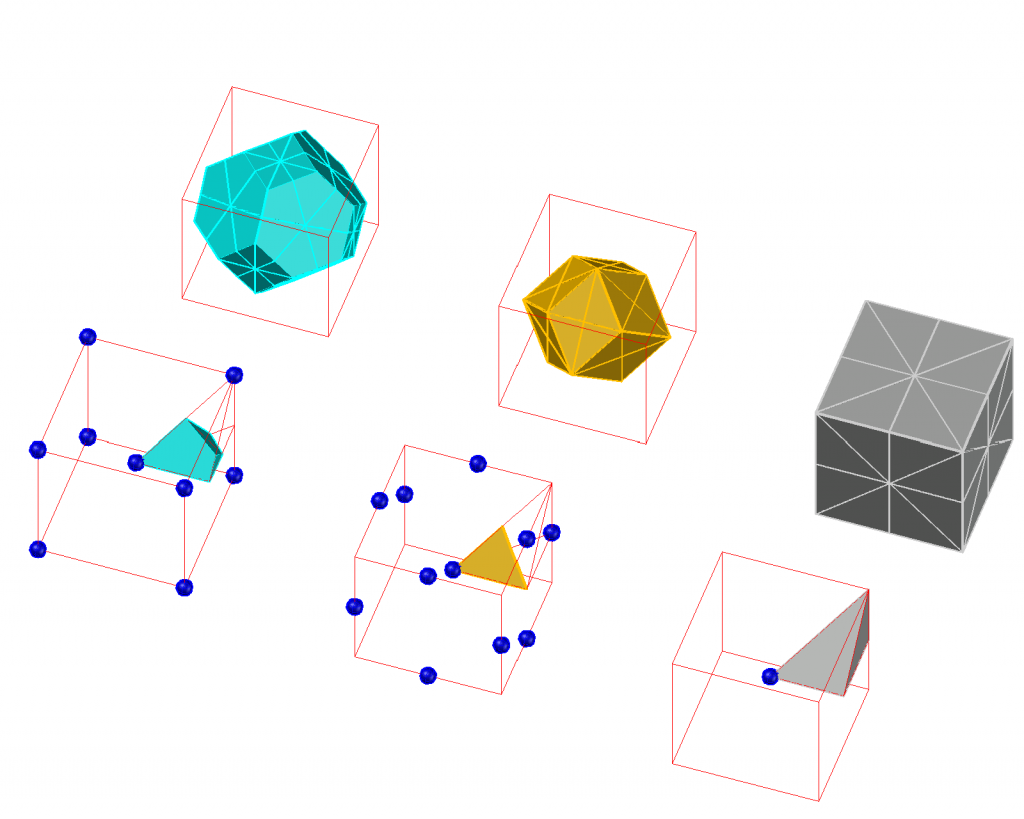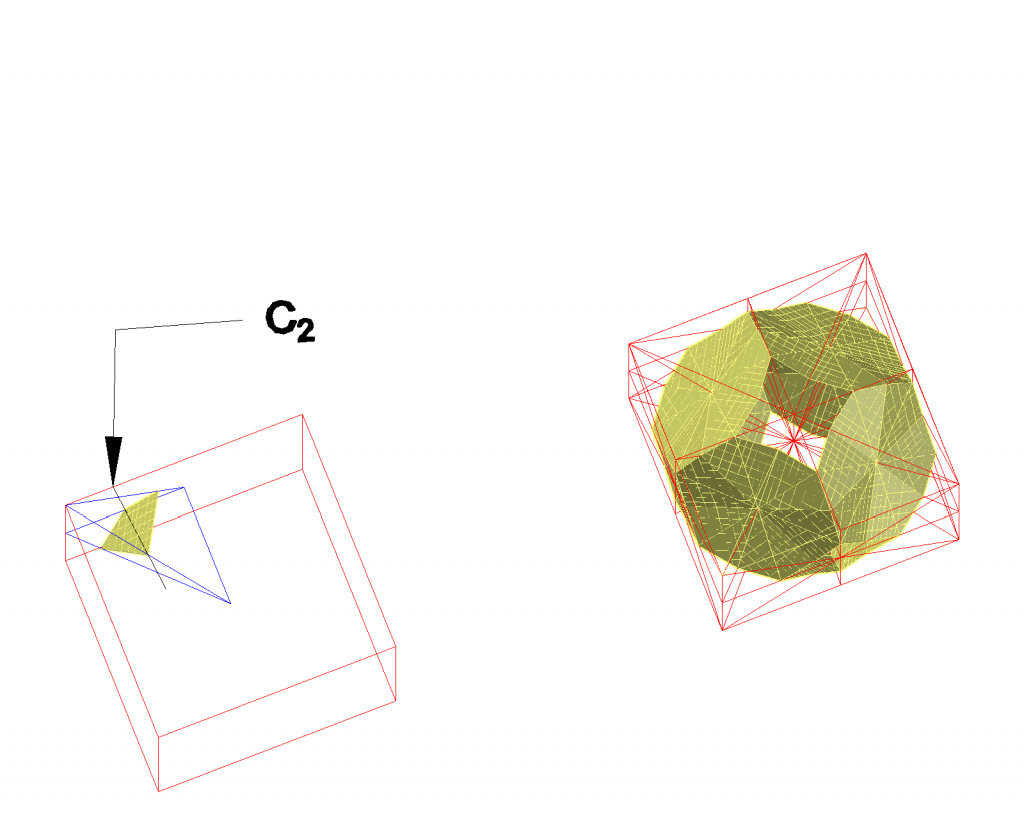# Space Filling Cubic Polyhedra.

The cubic honeycomb is the only regular honeycomb in Euclidian 3-space. There are only 5 space-filling polyhedra using translations only, called parallelohedra. Three of them have cubic symmetry. They are the Wigner-Seitz cells of the primitive, body-centered and face-centered cubic lattices (see fig. 1)Fig.1 Wigner Seitz cells of the body-centered, face-centered and primitivecubic honeycombs. In the lower half of the picture the corresponding basic polyhedra are shown from which the WS-cells may be obtained by applying all possible cubic mirror operations.

The cube can be divided into 48 tetrahedra by the mirror planes of the cubic system. It is in fact a first type Hill tetrahedron

The basic tetrahedron of the primitive cube can be divided into two identical halves for the body-centered cubic Wigner-Seitz cell, or truncated octahedron, and into four identical basic polyhedra for the face-centered cubic Wigner-Seitz cell, the rhombic dodecahedron.

The truncated octahedron is not the only space filling polyhedron on a body centered cubic lattice, because the basic tetrahedron of the primitive cube can be divided into identical halves in many different ways by a separation plane containing the twofold axis connecting the two halves (fig.2). Two of the polyhedra obtained in this way are the Hill tetrahedra type 2 and 3.Fig. 2 Left: Plane disecting the basic cubic tetrahedron containing a two-fold axis.Right: By applying all possible cubic mirror planes an infinite polytopic surface is obtained, separating two congruent infinite polyhedra.

All examples of the bcc spacefilling polyhedra shown in fig.3 are non-convex in contrast to the truncated octahedron, the WS cell.

The magenta object is the well-known stellated rhombic dodecahedron, also known as the Escher solid. The basic tetrahedron is a combination of two basic tetrahedra of the fcc Wigner Seitz cell . The separation plane contains the face center of the cube. Its basic tetrahedron is a Hill type 2 tetrahedron.

The grey object is a cubic cross. The separation plane contains a point halfway the cube edge and a face center.

The orange object is special, because the separation plane contains the cube center. Its basic tetrahedron is a Hill type 3 tetrahedron. The honeycomb can alternatively be built by the space filling star-shaped hexadecahedron ( in projection looking like a wind rose) shown in the upper left part of the picture. In the space filling tiling six of these stars have a body diagonal of the cube in common. These stars fit into the slots at the cube edges. In fig. 4 resin prints of the wind-rose-like objects are shown.